# How to use the LOGNORM.INV function in Excel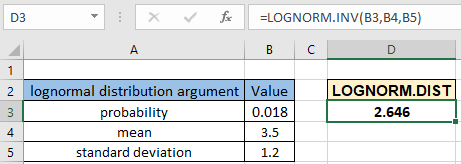In this article, we will learn How to use the LOGNORM.INV function in Excel.

What is the lognormal distribution function and inverse of lognormal distribution function ?

In Statistics, a log-normal (or lognormal) distribution is a continuous probability distribution of a random variable whose logarithm is normally distributed. A random variable which is log-normally distributed takes only positive real values. It is a convenient and useful model for measurements in exact and engineering sciences as well as medicine, economics and other fields, e.g. for energies, concentrations, lengths, financial returns and other amounts. So if the data has x1,x2, x3, ...etc values, then the lognormal distribution has ln(x1), ln(x2), ln(x3), ...etc values. Now our new random variable becomes new x1, new x2,..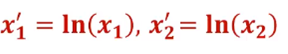So inversely we can say x1 = e^(new x1) as shown below,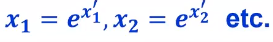Here

e is Euler's number ( value of e = 2.71828...).

Lognormal distribution is often applicable in time to repair equipment, time to fatigue failure or economic data, basically saying while getting the idea for maintenance things or values. Mathematically, we can calculate the probability for lognormal distribution function using the formula stated below.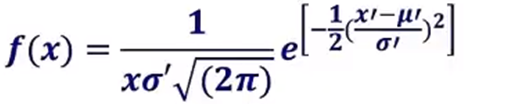Here

e is Euler's number ( value of e = 2.71828...).

x value at which inverse of lognormal distribution is evaluated

x , mean , std  is the value, mean and standard deviation for the normal distribution

x' , mean', std' is the value, mean and standard deviation for the lognormal distribution.

We calculate the probability value for x value using the LOGNORM.DIST function. But if you want to find out the value x from the lognormal probability value using the mean and standard deviation.

This formulation seems complex to formulate in Excel. So Excel provides a built-in statistical LOGNORM.INV function to work as an inverse of lognormal distribution calculator.

LOGNORM.INV Function in Excel

LOGNORM.INV function built in statistical function returns the x value for the probability of lognormal distribution. It takes the 3 arguments. Let's understand these arguments one by one listed below with the function syntax.

LOGNORM.INV Function syntax:

 =LOGNORM.INV(probability, mean, std_dev)

probability : probability value for the distribution

mean : mean of inverse for lognormal distribution

std_dev : standard deviation for inverse of lognormal distribution

Example :

All of these might be confusing to understand. Let's understand how to use the function using an example. Here we have probability value, mean value and standard deviation value. We need to calculate the x value for the inverse of lognormal distribution function.

Use the formula:

 =LOGNORM.INV ( B3, B4, B5 )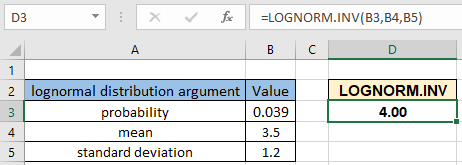The x value for the lognormal distribution comes out 4for the value probability value 0.039 or 3.9% with mean 3.5 and standard deviation 1.2.

You can perform the inverse of this function i.e. you can find the probability value using the x value, mean value and standard deviation value for the lognormal distribution using the LOGNORM.DIST function. Use the cumulative argument as TRUE to get the x value value.

Here are all the observational notes using the LOGNORM.INV function in Excel
Notes :

1. The function only works with numbers. If any argument other than cumulative is non numeric, the function returns #VALUE! error.
2. The function returns #NUM! Error.
1. If argument prob < 0 or > 1
2. If argument mean <= 0
3. If argument std_dev < 0
3. Value in decimal and value in percentage id the same value in Excel. Convert the value to percentage, if required.
4. You can feed the arguments to the function directly or using the cell reference as explained in the example.

Hope this article about How to use the LOGNORM.INV function in Excel is explanatory. Find more articles on statistical formulas and related Excel functions here. If you liked our blogs, share it with your friends on Facebook. And also you can follow us on Twitter and Facebook. We would love to hear from you, do let us know how we can improve, complement or innovate our work and make it better for you. Write to us at info@exceltip.com.

Related Articles :

How to use Excel NORM.DIST Function : Calculate the Z score for the normal cumulative distribution for the pre specified values using the NORMDIST function in Excel.

How to use Excel NORM.INV Function : Calculate the inverse of Z score for the normal cumulative distribution for the pre-specified probability values using the NORM.INV function in Excel.

How to use Excel F.TEST Function in Excel : The F.TEST Function is used to calculate F statistic of two samples in excel internally and returns the two tailed probability of the F statistic under Null Hypothesis.

How To Use Excel T.TEST Function in Excel : The T.TEST function is used to determine the confidence of an analysis in Excel. Mathematically, it is used to know if the mean of the two samples are equal or not. T.TEST function is used to accept or reject the null hypothesis.

How to use the DEVSQ Function in Excel : DEVSQ function is a built-in statistical function to calculate the sum of squared deviations from the mean or average of the given distribution provided.

How to calculate Standard Deviation in Excel : To calculate the standard deviation we have different functions in Excel. The standard deviation is the square root of the variance value but It tells more about the dataset than variance.

How to use the VAR function in Excel : Calculate the variance for the sample dataset in excel using the VAR function in Excel.

Popular Articles :

How to use the IF Function in Excel : The IF statement in Excel checks the condition and returns a specific value if the condition is TRUE or returns another specific value if FALSE.

How to use the VLOOKUP Function in Excel : This is one of the most used and popular functions of excel that is used to lookup value from different ranges and sheets.

How to use the SUMIF Function in Excel : This is another dashboard essential function. This helps you sum up values on specific conditions.

How to use the COUNTIF Function in Excel : Count values with conditions using this amazing function. You don't need to filter your data to count specific values. Countif function is essential to prepare your dashboard.

Terms and Conditions of use

The applications/code on this site are distributed as is and without warranties or liability. In no event shall the owner of the copyrights, or the authors of the applications/code be liable for any loss of profit, any problems or any damage resulting from the use or evaluation of the applications/code.